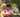### Merge Sort

January 18, 2020

Merge Sort Algorithm in JavaScript

The mergeSort() will divide the given array into smaller arrays in every iteration of the recursive call. Use the middle index and split the array into left and right arrays. The merge() will sort all the elements in the left and the right using while loop. Finally, use concat() to include the remaining ordered elements.

``````// Merge the two arrays: left and right
const merge = (left, right) => {
let resultArray = [], leftIndex = 0, rightIndex = 0;

while (leftIndex < left.length && rightIndex < right.length) {
if (left[leftIndex] < right[rightIndex]) {
resultArray.push(left[leftIndex]);
leftIndex++; // move left array cursor
} else { // left > right
resultArray.push(right[rightIndex]);
rightIndex++; // move right array cursor
}
} // looping stops when one side reaches the last element

if(leftIndex < left.length) return resultArray.concat(left.slice(leftIndex))
return resultArray.concat(right.slice(rightIndex))
}

function mergeSort (A) {
if (A.length <= 1) return A

// In order to divide the array in half, we need to figure out the middle
const middle = Math.floor(A.length / 2);

// This is where we will be dividing the array into left and right
const left = A.slice(0, middle);
const right = A.slice(middle);

// Using recursion to combine the left and right
return merge( mergeSort(left), mergeSort(right) );
}

console.log("mergeSort([10, -1, 2, 5, 0, 6, 4, -5]): ", mergeSort([10, -1, 2, 5, 0, 6, 4, -5]))``````Posted by Tai Lu ( 呂台生 ) who's building useful things.You should follow him on Twitter